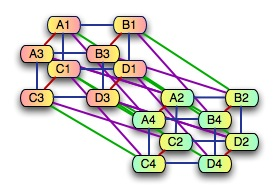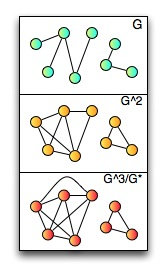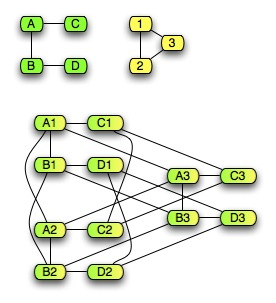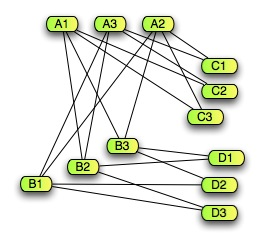# Powers and Products of GraphsOften, we use graphs as a structured representation of some kind of information. Many interesting problems involving the things we’re representing can then by described in terms of operations over
graphs. Today, I’m going to talk about some of the basic operations that we can do on graphs, and in later posts, we’ll see how those operations are used to solve graph-based problems.The easiest thing to describe is exponents of a graph. Given a graph G=(V,E), for
an integer n, Gn is a graph with the same vertices as G, and with edges between
two vertices v and w if and only if there is a path between v and w that contains fewer
than n edges. For a finite graph, after a finite number of steps, increasing the exponent won’t
change the graph – the graph contains edges between any nodes that will ever be connected. That
graph is called the closure of G, written G*. If G is an undirected,
connected graph, then G* will be Kx where x is the number of vertices in G.
For an example of exponents and closure, see the graph to the left, which shows a graph G, G2, and G3. For this graph, G* is the same as G3.

The next most common operation – or in the case of graphs, family of operations – is product. For graphs, there are a variety of different kinds of graph products: cartesian product, lexicographic (or ordered) product, tensor product, and strong product are the most common ones. All of them have the same vertex sets, but they each have different ways of defining the edges.

In all of the graph products, the set of vertices of two graphs G=(V,E) and H=(W,F) is
V×W – that is, the set of pairs of a vertex from V and a vertex from W. So, if G is
a graph with vertices{A,B,C,D}, and H is a graph with vertices {1,2,3}, then the set of vertices in the product of G and H
is {(A,1),(A,2),(A,3),(B,1),(B,2),(B,3),(C,1),(C,2),(C,3),(D,1),(D,2),(D,3),}.The cartesian product is the simplest one. For any two vertices (v,w) and (v’,w’) in the cartesian product of G and H, then there is an edge between (v,v’) and (w,w’) if and only if v=v’and (w,w’) is an edge in H, or w=w’ and (v,v’) is an edge in G. That sounds kind of frightening, but it’s really
pretty straightforward, as you can see by looking at an example. It’s a straightforward version of
the basic idea of cartesian product in sets or topologies. The idea of it is basically
that for any vertex in G, there’s a copy of H (with edges), and the copies are connected in the structure of G. For example, to the right is a simple pair of graphs – one with four vertices, and
the second one with three. Beneath it is a drawing of the cartesian product of the two graphs. The cartesian graph product is commutative and associative, and it’s normally written as a box: A[]B. (Not quite the right thing, but I couldn’t find an HTML entity for a hollow square.)

(note: there was an error in the original version of the above paragraph, mixing the v’s and w’s.)

One thing that I think is rather cool about the cartesian product is that it preserves hypercubes. A hypercube is, basically, what you get if you take an N-dimensional cube, make each corner a vertex, and then put edges between two vertices in the graph if there’s a cube edge between the corners corresponding to the two corners. What it means to preserve hypercubes is: if you take the cartesian product of two hypercubes, the result is also a hypercube. The product of a square (a 2-cube) and a line (a 1-cube) is a common three-dimensional cube. The product of two squares is a tesseract – a 4-cube. There’s an illustration of the product of two squares laid out and colored as best I can to make the tesseract structure clear up in the header of this post.The tensor product of two graphs has a set of edges that’s actually easier to understand in the
definition, but it’s a tad harder to understand what it means. The edges are a subset of the edges in
the cartesian product. In the tensor product, there is an edge between (v,w) and (v’,w’) if and only
if (v,v’) is an edge in G, and (w,w’) is an edge in H. You can see it as a kind of a join of the edges
in G and H – an edge between paired vertices only exists if there is an edge between both of the
vertices that make up the pair in the original graph. To the left, there’s an example of the tensor
product for the same pair of graphs as the cartesian product example. The tensor product is also the product in the category of graphs – which, in some sense, makes it the most fundamental form of the product. (Just to make things confusing, the cartesian product of graphs is not not a categorial product, but a tensor.) Tensor product is associative and commutative, and is written using the usual product notation: A×B. Tensor product doesn’t preserve hypercubes, but it does preserve bipartiteness: the tensor product of two bipartite graphs is a bipartite graph.

The lexicographic product looks strange in its definition. But the idea of it is actually
relatively simple. If you use ordered graphs in the lexicographic product, it actually makes
a lot of sense. Treat each graph as the definition of a partial order over its sets of vertices. Then
the lexicographic product is a graph which defines a partial order of the product of the two sets
which is consistent with the orderings over the two member sets. To be precise about it, the
lexicographic product of two graphs has an edge between (v,w) and (v’,w’) if and only if G contains an
edge (v,v’), or v=w and (w,w’) is an edge in H. Lexicographic product is (obviously) not commutative
– the order in which graphs are combined using lexicographic product is crucial. It’s
generally written like scalar product A*B; it’s also often called graph composition. For
our example graph, the lexicographic product ends up looking the same as the cartesian product.

(Note: in the original version of the above paragraph, I wrote that lexicographic product
was not associative. Of course, it is – if it weren’t, then the result of multiple lexicographic products
of ordered graphs couldn’t possibly be consistent with the orderings of the element graphs. I don’t know what I was thinking when I wrote that; it’s a dumb error.)

## 27 thoughts on “Powers and Products of Graphs”

1.Xanthir, FCD

Typo in the cartesian product definition:
“then there is an edge between (v,w) and (v’,w’) if and only if v=v’ and (w,w’) is an edge in H, or w=w’ and (v,v’) is an edge in G.”

2.Isabel

Is this definition of a power of a graph standard? I think I’m bothered by it because G^2 isn’t the product of G with G, which isn’t totally necessary but seems like a nice thing to have.

3.Mark C. Chu-Carroll

Thanks for catching that Xanthir. It’s hard to type in multiple subtly different definitions like this without making a mistake…

4.Mark C. Chu-Carroll

Isabel:
Yes, that’s the standard definition of graph exponent. It’s annoying that it’s disconnected from the graph product, but that’s the common usage. The “other” exponents – that is, the multiplicative ones – are not used very much.

5.Jonathan Vos Post

Ji Li and I gave different definitions of “prime graph.” His was in terms of Cartesian products of graphs. Mine was with categorical products of graphs.
A nice table of the different kinds of graph products is at:
http://mathworld.wolfram.com/GraphProduct.html

6.Pinky

All math are good depending on axioms.

7.William

Typo in the lexicographic definition. Should be “the lexicographic product of two graphs has an edge between (v,w) and (v’,w’) if and only if G contains an edge (v,v’), or v=v’ and (w,w’) is an edge in H.”

8.Torbjörn Larsson, OM

The product of two squares is a tesseract – a 4-cube.

Maybe I am imagining things, but it looks like the tesseract is also a cartesian product of a cube and a line?

The tensor product … The edges are a subset of the edges in the cartesian product.

Except that it doesn’t look that way in the figure to me, but as the complement of the cartesian edges.
Perhaps a typo in the definition, or is it now inconsistent with the previous corrected one? “an edge between (v,v’) and (w,w’) if and only if (v,v’) is an edge in G, and (w,w’) is an edge in H” would perhaps make the edges a subset of the cartesian edges. I’m giving up on the figure though. 😛

9.Torbjörn Larsson, OM

Hmm. Or rather the original definition may be consistent with Xanthir’s and William’s corrections, when they are introduced. Assuming subset is desired, in which case I still don’t get the accompanying figure.

10.tom

Are you sure the set of edges from the tensor product is a subset of the edges from the tensor product? It looks like a disjoint set to me.
By the way, did anyone manage to find a valid sequence of contractions to take the 20-vertex snark from the 8th of July into Petersen’s graph? I can’t do it without contracting non-adjacent verticies, which didn’t seem to be allowed.
Each vertex in each graph has exactly 3 edges, but contraction tends to increase the number of edges per vertex. Vertex edge-counts only go down again when you start to ‘squash-out’ cycles. I was hoping to be able to work out the contraction sequence by examining the patterns of cycles in each graph, but that hasn’t helped me yet.
Any hints?

11.Matías Giovannini

The lexicographic product is indeed associative. Please see my comment on http://programming.reddit.com/info/28yk7/comments/c28zls for a proof.

12.Anthony L

Small white square should be &# 9633; (without the space)

13.JBL

Torbjörn wrote:
“Maybe I am imagining things, but it looks like the tesseract is also a cartesian product of a cube and a line?”
Of course: if L is a line (a graph with two vertices and one edge) then a square is (L x L) and a cube is (L x L x L). Then (L x L) x (L x L) = (L x L x L) x L by the associativity of the Cartesian product, so the product of two squares is the same as the product of a cube and a line.

14.JBL

In case it wasn’t clear, I was using ” x ” for the Cartesian product in the above post.
Isabel: the graph exponent notation that Mark introduced is particularly useful when dealing with walks on graphs, because a single step in G^n is equivalent to n steps in G. So, if you want to know something about, say, random walks on a graph, that graph exponent is very helpful. It’s also related to powers of the adjacency matrix, which may help explain the notation. In contexts where the chance of confusion is low, I have no doubt that mathematicians will use the exponent notation when dealing with different types of graph products.

15.Vorn

The “graph exponent” makes a certain amount of sense to me – it turns out that (Ga)b = Gab (go ahead, try it – Ga gets you every pair of nodes a steps apart joined; so (Ga)b gets you every pair of nodes a×b edges apart joined, because you can take b a-length edges, so you get Gab), and that is one of the usual exponent identities.
Vorn

16.Anonymous

also, □ □ “White Square”

17.milan_va

From what I know, there should only be 4 edges adjacent to any vertex of a tesseract. It seems to me that none of the violet lines should be there, in the first image.
Perhaps they are what makes the image so confusing. A tesseract is actually quite a simple thing.
I appreciate the effort that has been put into the painting, though 😉

18.Torbjörn Larsson, OM

JBL:
Yes, formally. What you are saying is that the graph cartesian product behaves exactly as the the usual one. Thanks for helping out.

19.bill

Does the idea of power extend to a root (a graph G s.t. G^2 is the target graph), or a minimal generator (the minimal graph such that G = M^k)?

20.Xanthir, FCD

bill:
I see no reason why it shouldn’t. However, the root would not be well-defined – there will usually be multiple graphs that all go to the same graph when squared.
JBL:
In your response to Isabel, you say that a step in G^n is equivalent to n steps in G. Shouldn’t that be “n or less” steps in G? There are some fairly simple cases where a single step in G^n is *impossible* to reproduce with n steps in G. For example, given G as the square (a ring of 4 elements), G^2 is maximally connected. And yet it’s impossible to go from one vertex to an adjacent vertex in G in 2 steps, despite the fact that there is an edge connecting them in G^2.

21.JBL

Xanthir: Yes, my wording was sloppy. There are some reasons one might prefer the definition I did give (it’s easy to get from the definition I gave to Mark’s definition but not vice-versa, and the definition I gave relates more immediately to the adjacency matrix of the graph), but it seems that the definition Mark gave is very much standard.

22.JBL

bill: Mathworld says
“Mukhopadhyay (1967) has considered “square root graphs,” whose square gives a given graph G (Skiena 1990, p. 253).”
and gives the reference
Mukhopadhyay, A. “The Square Root of a Graph.” J. Combin. Th. 2, 290-295, 1967.

23.Anonymous

At the start of your second paragraph you write: “The easiest thing to describe is exponents of a graph. Given a graph G=(V,E), for an integer n, Gn is a graph with the same vertices as G, and with edges between two vertices v and w if and only if there is a path between v and w that contains fewer than n edges.”
But your illustration seems to show that there’s an edge between vertices in Gn if there’s a path between v and w that contains n or fewer edges. So which is it?

24.Antendren

n or fewer. After all, we want G^1 = G.

25.Jonathan Vos Post

Wikipedia’s “Graph Operations” usefully mentions:
* Power of graph: The k-th power G^k of a graph G is a supergraph formed by adding an edge between all pairs of vertices of G with distance at most k. A second power of a graph is also called a square.
[Caveat: In mathematics and physics, the word supergraph has two main meanings:
(1) If A is a subgraph of B, then B is said to be a supergraph of A
(2) In the context of particle physics, a supergraph is a Feynman diagram that calculates scattering amplitudes in a supersymmetric theory using the advantages of the superspace formalism].
Graph products based on the Cartesian product of the vertex sets:
o Cartesian product of graphs; It is a commutative and associative operation (for unlabelled graphs).
o Lexicographic product of graphs (also called graph composition); It is noncommutative, non-associative
o Strong product of graphs
o Tensor product of graphs, also called direct product, categorical product, cardinal product, or Kronecker product. It is a commutative operation (for unlabelled graphs)
o Zig-zag product of graphs Let [N] denote the set of integers from 1 to N. It is supposed that k-regular graphs used in the definition below are k-edge colored, i.e., their edge sets are partitioned into k perfect matchings. For each color i and a vertex v let v[i] denote the neighbor of v along the edge colored with color i. Let G1 be a D1-regular graph on [N1] and let G2 be a D2-regular graph on [D1]. Then the zig-gag product H is a graph with vertex set [N1] × [D1], where for all n in [N1], d in [D1], and i,j, in [D2], the vertex (n, d) is connected to (n[d[i]], d[i][j]). This definition is used in construction of expander graphs.
* Other graph operations called “products”:
o Rooted product of graphs. It is noncommutative, non-associative
o Corona product or simply corona of G1 and G2, defined by Frucht and Harary is the graph which is the disjoint union of one copy of G1 and |V1| copies of G2 (|V1| is the number of vertices of G1) in which each vertex of the copy of G1 is connected to all vertices of a separate copy of G2.
Nebesky, Ladislav, On the existence of a 3-factor in the fourth power of a graph. With a loose Russian summary. also page 209
Casopis Pest. Mat. 105 (1980) 204-207 05C70
Rajeev Motwani, Madhu Sudan, Computing Roots of Graphs is Hard (1994)
Abstract: The square of an undirected graph G is the graph G 2 on the same vertex set such that there is an edge between two vertices in G 2 if and only if they are at distance at most 2 in G. The k’th power of a graph is defined analogously. It has been conjectured that the problem of computing any square root of a square graph, or even that of deciding whether a graph is a square, is NP-hard. We settle this conjecture in the affirmative…
Alon, Noga; Lubetzky, Eyal, “Graph powers, Delsarte, Hoffman, Ramsey and Shannon”
arXiv:math/0608013
August 2006
Abstract:
The k-th p-power of a graph G is the graph on the vertex set V(G)^k, where two k-tuples are adjacent iff the number of their coordinates which are adjacent in G is not congruent to 0 modulo p. The clique number of powers of G is poly-logarithmic in the number of vertices, thus graphs with small independence numbers in their p-powers do not contain large homogenous subsets. We provide algebraic upper bounds for the asymptotic behavior of independence numbers of such powers, settling a conjecture of Alon and Lubetzky up to a factor of 2. For precise bounds on some graphs, we apply Delsarte’s linear programming bound and Hoffman’s eigenvalue bound. Finally, we show that for any nontrivial graph G, one can point out specific induced subgraphs of large p-powers of G with neither a large clique nor a large independent set. We prove that the larger the Shannon capacity of \$bar{G}\$ is, the larger these subgraphs are, and if G is the complete graph, then some p-power of G matches the bounds of the Frankl-Wilson Ramsey construction, and is in fact a subgraph of a variant of that construction.

26.bill

JBL: Thanks.

27.Roli Eballe

Hello everybody,
I would like to know whether the independence number of the tensor product of graphs G and H can be computed exclusively from the independence number of the factors. Has there been any result to this effect?